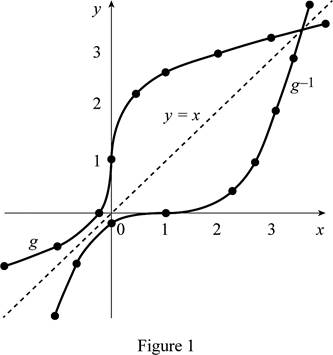# To state: The value of g ( 2 ) .### Single Variable Calculus: Concepts...

4th Edition
James Stewart
Publisher: Cengage Learning
ISBN: 9781337687805### Single Variable Calculus: Concepts...

4th Edition
James Stewart
Publisher: Cengage Learning
ISBN: 9781337687805

#### Solutions

Chapter 1, Problem 2RE

(a)

To determine

## To state: The value of g(2).

Expert Solution

Solution:

The value of g(2) is 3.

### Explanation of Solution

Let y=g(x) be the function.

From the given graph, it is noticed that when x=2, then the curve approaches to 3.

That is, g(2)=3.

(b)

To determine

Expert Solution

### Explanation of Solution

The function is one-to-one if it satisfies the horizontal line test.

Horizontal line test states that, if the horizontal line intersects the curve atmost once then the function g is one-to-one.

From the given graph, it is noticed that no horizontal line intersects the graph more than once. So, it satisfies the horizontal line test.

Hence, the function f is one-to-one.

(c)

To determine

### To estimate: The values of g−1(2).

Expert Solution

Solution:

The values of g1(2) is approximately equal to 2.5.

### Explanation of Solution

Let y=g(x) be the function, then g1(y)=x.

From the given graph, it is noticed that the value of g(x)=2 when x=2.5.

Then, it can be implied that g1(2)2.5

When y=2, then g1(2)2.5.

(d)

To determine

### To estimate: The domain of g−1.

Expert Solution

Solution:

The domain of g1 is [1,3.5].

### Explanation of Solution

It is known that the domain of g1 is the range of g and the range of g1 is the domain of g.

From the given graph, it is observed that the range of g is [1,3.5].

Therefore, the domain of g1 is [1,3.5].

(e)

To determine

Expert Solution

### Explanation of Solution

Graph:

The graph of g1 is obtained by taking the reflection of g about the line y=x. Sketch the graph of g1 as shown below in Figure 1.From Figure1, it is observed that g is the reflection of g1 about the line y=x.

### Have a homework question?

Subscribe to bartleby learn! Ask subject matter experts 30 homework questions each month. Plus, you’ll have access to millions of step-by-step textbook answers!